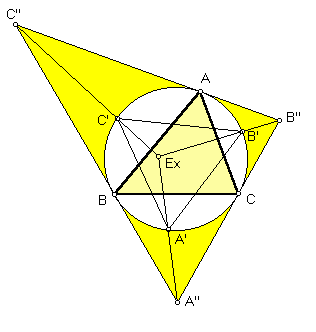# EXETER POINT

 Discovered to lie on the Euler line at a computers-in-mathematics workshop at Phillips Exeter Academy in 1986, the Exeter point is the center of perspective of the tangential triangle (formed by the tangents to the circumcircle at the vertices A, B, C) and the circummedial triangle (whose vertices are points of intersection of the medians with the circumcircle). Trilinears for the Exeter point are a (b^4 + c^4 - a^4) : b (c^4 + a^4 - b^4) : c (a^4 + b^4 - c^4).(For a quick lesson on trilinears, click on TRILINEAR COORDINATES.)
Triangle Centers